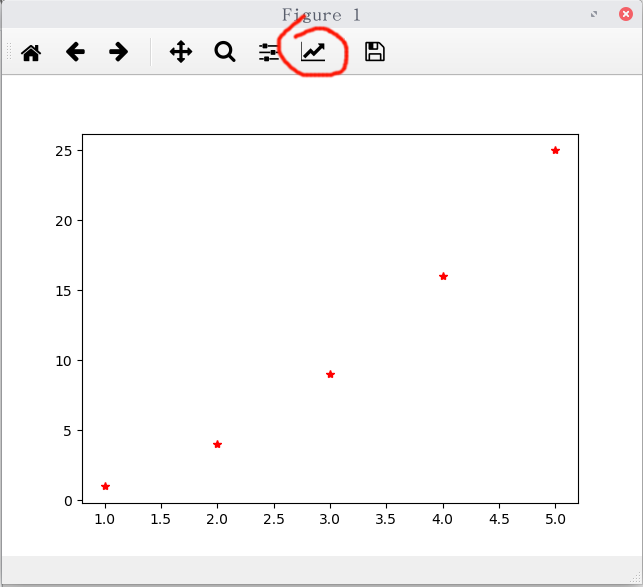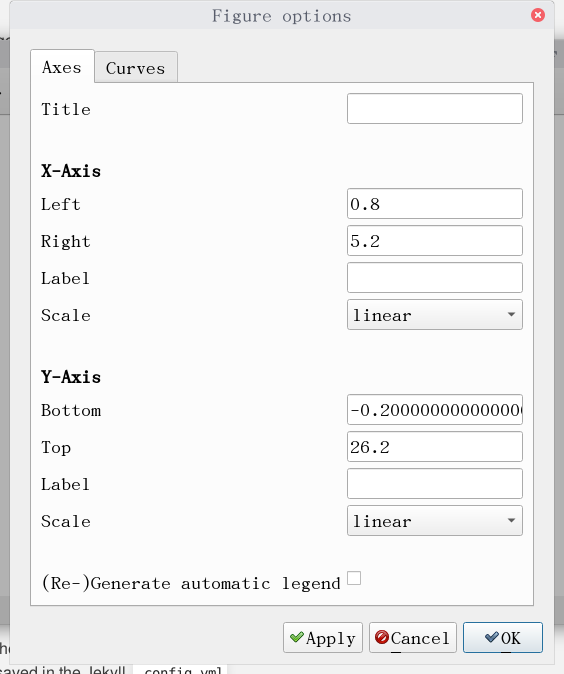# some experiences of using python to deal with data in physics experiment of USTC.

There are many modules in python, such as matplotlib, numpy, scipy, sympy and so on. So, you can do many things with python.

Some times, there are many data to process in physics experiment of USTC, so, why not use python to help you?

`ipython` is a good interactive shell of python, it have syntax highlighting, auto complete, auto indentation and so on, and it doesn’t occupy much.

You can record your data in list, and write into a script at first, and when you want to process data, you can use command

``````cat data.py - | ipython
``````

to load the data and enter ipython. Or, you can also record data only when you want to process data, and ipython have commands such as `%save` to help you save the data.

And then, I will introduce some modules for you to use.

• matplotlib: It’s a good module to plot functions.
``````  import matplotlib.pyplot as plt

x = [1, 2, 3, 4, 5]
y = [z ** 2 for z in x]
plt.plot(x, y, 'r*')
plt.show()
``````

and ipython will output:you can change the title of graphics, and change the label of axies by clicking the button which surrounded by red circle, and it will pop up a form:so you can edit the title and others after plot generated and don’t need to adjust it by typing codes. `'r*'` is the format of line, `*` is to use ‘*’ as points, and `r` is to use color red.

• numpy: It’s a good module for scientific computing
``````  y = [z ** 2 for z in x]
``````

in last paragraph can be replaced by

``````  import numpy as np
...
y = np.square(x)
``````

numpy can calculate array directly and if you want to plot a function:

``````  import matplotlib.pyplot as plt
import numpy as np

x = np.linspace(0, 100, 1000)
y = np.sqrt(x)
plt.plot(x, y, 'r')
plt.show()
``````

and it will output:numpy can also do calculate of matrix, even solve the equation set.

• sympy: a good symbol calculating module use it to solve the equation and others, even do some calculus calculating.
• derivative:
``````  from sympy import Symbol, diff, exp

x = Symbol('x')
diff(exp(x**2), x)
Out: 2*x*exp(x**2)
``````
• integrals:
``````  from sympy import Symbol, integrate, cos, exp, oo

x = Symbol('x')
integrate(cos(x), x)
Out: sin(x)
integrate(exp(-x), (x, 0, oo))
Out: 1
``````
• … you can use it to derive the function of uncertainty.

and you can also use `def`, `for` or other key words to simplify your data processing.

All in all, python doesn’t have much disadvantage to other scientific calculating software, and it even have more functions, it is very useful in data processing.